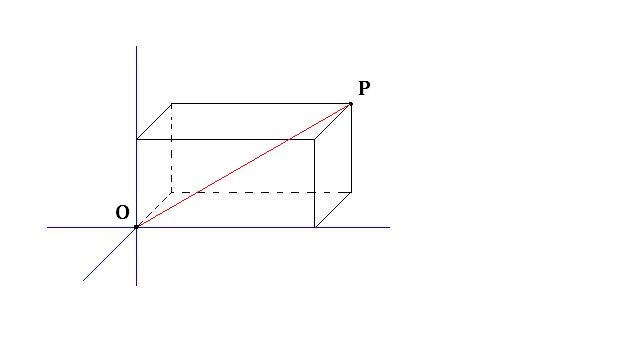Box in the Air (Part 2)

Geometry Level 2The point $O$ is the origin in the $xyz$ coordinates, and the point $P$ is the vertex of a cuboid, as shown above. The length of $OP$ is 7 while the three dimensions are all integers, where the length is the product of the width and the height of the cuboid.

What is the volume of the cuboid?

×